# High School Math : Polynomials

## Example Questions

### Example Question #1 : Understanding Zeros Of A Polynomial

Factor the polynomial if the expression is equal to zero when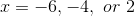.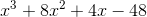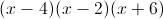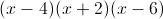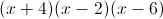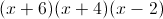Explanation:

Knowing the zeroes makes it relatively easy to factor the polynomial.

The expression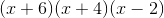fits the description of the zeroes.

Now we need to check the answer.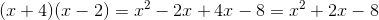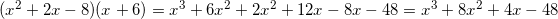We are able to get back to the original expression, meaning that the answer is.

### Example Question #11 : Pre Calculus

A polyomial with leading term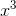has 6 as a triple root. What is this polynomial?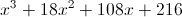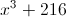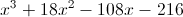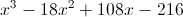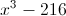Explanation:

Since 6 is a triple root, and the degree of the polynomial is 3, the polynomial is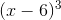, which we can expland using the cube of a binomial pattern.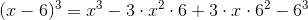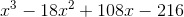### Example Question #4 : Express A Polynomial As A Product Of Linear Factors

A polyomial with leading termhas 5 and 7 as roots; 7 is a double root. What is this polynomial?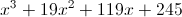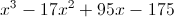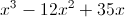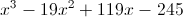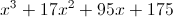Explanation:

Since 5 is a single root and 7 is a double root, and the degree of the polynomial is 3, the polynomial is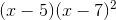. To put this in expanded form: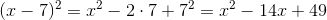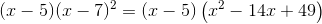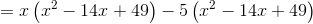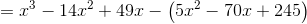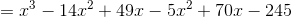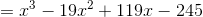### Example Question #3 : Understanding Zeros Of A Polynomial

What are the solutions to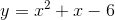?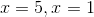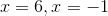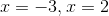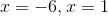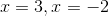Explanation:

When we are looking for the solutions of a quadratic, or the zeroes, we are looking for the values ofsuch that the output will be zero. Thus, we first factor the equation.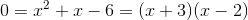Then, we are looking for the values where each of these factors are equal to zero.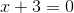implies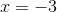and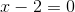impliesThus, these are our solutions.

### Example Question #1 : Finding Zeros Of A Polynomial

Find the zeros of the following polynomial: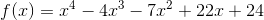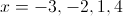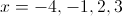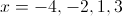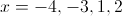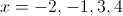Explanation:

First, we need to find all the possible rational roots of the polynomial using the Rational Roots Theorem: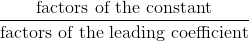Since the leading coefficient is just 1, we have the following possible (rational) roots to try:

±1, ±2, ±3, ±4, ±6, ±12, ±24

When we substitute one of these numbers for, we're hoping that the equation ends up equaling zero. Let's see ifis a zero: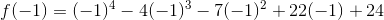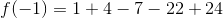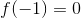Since the function equals zero whenis, one of the factors of the polynomial is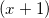. This doesn't help us find the other factors, however. We can use synthetic substitution as a shorter way than long division to factor the equation.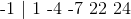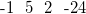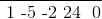Now we can factor the function this way:We repeat this process, using the Rational Roots Theorem with the second term to find a possible zero. Let's try: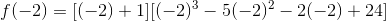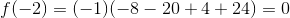When we factor using synthetic substitution for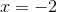, we get the following result: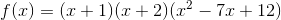Using our quadratic factoring rules, we can factor completely: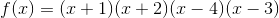Thus, the zeroes ofare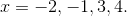### Example Question #1 : Simplifying Polynomial Functions

Simplify the following polynomial: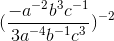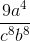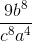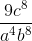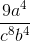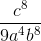Explanation:

To simplify the polynomial, begin by combining like terms: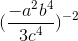### Example Question #21 : Pre Calculus

Simplify the following polynomial function: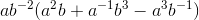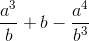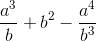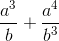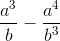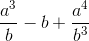Explanation:

First, multiply the outside term with each term within the parentheses: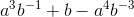Rearranging the polynomial into fractional form, we get:### All High School Math Resources